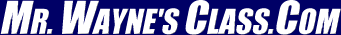IntroductionThis text is meant to accompany class discussions. It is not everything there is to know about uniform circular motion. It is meant as a  prep for class. More detailed notes and examples are given in the class notes, presentations, and demonstrations (click here.)

 Page Description 0 Objectives 1 Skill: Finding the slope of a straight line 1 Skill: Finding the units of slope 2 Skill: Finding the slope from a curve 3 Position vs Time Graphs and the slope 4 Velocity vs Time Graphs and the slope 5 Acceleration vs Time Graphs and the slope 6 Jerk 7 Skill: Finding the Area on a Graph 7 Using Integration to Find More 8 Summary Quiz (10 questions)
Objectives

Students should be able to:
1       In a sentence define (Much of this should be review.)

 vector (give examples too) scalar (give examples too) displacement distance speed velocity acceleration jerk

2       Mathematically describe
instantaneous velocity on a graph
Calculate the slope and the units from a straight line
Calculate the clope and the units from a pointon a curve.

Kinematics & Graphing:
3       From a position vs time graph be able to answer questions about velocity
Example:
a        Find the position at a given time
b       Calculate the displacement
c        Find instantaneous velocity at a given time
d       Identify regions, line segments, of constant velocity
e       Identify regions, line segments, of positive velocity
f        Identify regions, line segments, of negative velocity
g       Identify regions, line segments, of acceleration
h       Identify the units of velocity or acceleration from the graph
i        Determine regions of positive or negative acceleration
j        Determine regions where the acceleration is zero

4       From a velocity vs. time graph be able to answer questions about acceleration and displacement
Example:
a        Find instantaneous velocity
b       Find instantaneous acceleration
c        Find displacement
d       Identify regions, line segments, of positive displacement
e        Identify regions, line segments, of negative displacement
f        Identify regions, line segments, of constant acceleration
g       Identify regions, line segments, of changing acceleration
h       Identify regions, line segments, of positive acceleration
i        Identify regions, line segments, of negative acceleration
h       Determine the units of displacement, velocity and acceleration from this graph
j        Identify regions of constant velocity and changing velocity
k        Identify regions of constant acceleration and changing acceleration

5      From an acceleration vs. time graph be able to answer questions about velocity
a        Find instantaneous jerk
b       Identify regions, line segments, of positive change in velocity
c        Identify regions, line segments, of negative change in velocity
d       Identify regions of changing jerk
e        Identify regions of constant jerk
f        Identify regions of changing acceleration
g       Identify regions of constant acceleration
h       Determine the units of velocity from an “a vs t” graph
i        Determine the units of jerk from an “a vs t” graph
j        Calculate the change in velocity

6        From a described situation draw either the
a        displacement vs. time graph or,
b       a velocity vs. time graph.

7      From an acceleration vs. time graph be able to answer questions about velocity
a        Find instantaneous acceleration
b       Find the change in velocity
c        Calculate the instantaneous jerk
d       Determine regions of constant acceleration
e        Determine regions of changing acceleration

ResourcesThese are questions that you need to know the answers to when entering the classroom.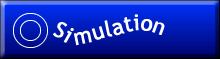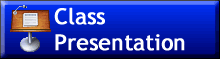This is the first presentation. It contains definitions, how to find slopes of a straight line and at a point on a curve. It also begines to developthe mneumonic for remembering this stuff.This presentation goes over more about tangent lines.This presentation covers how to calculate the "area" between the curve and the axis as weel as what that means.Summary of the mnumonic study aide with some examples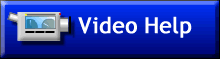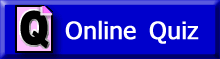Online Quiz #1Online Quiz #2Online Quiz #3